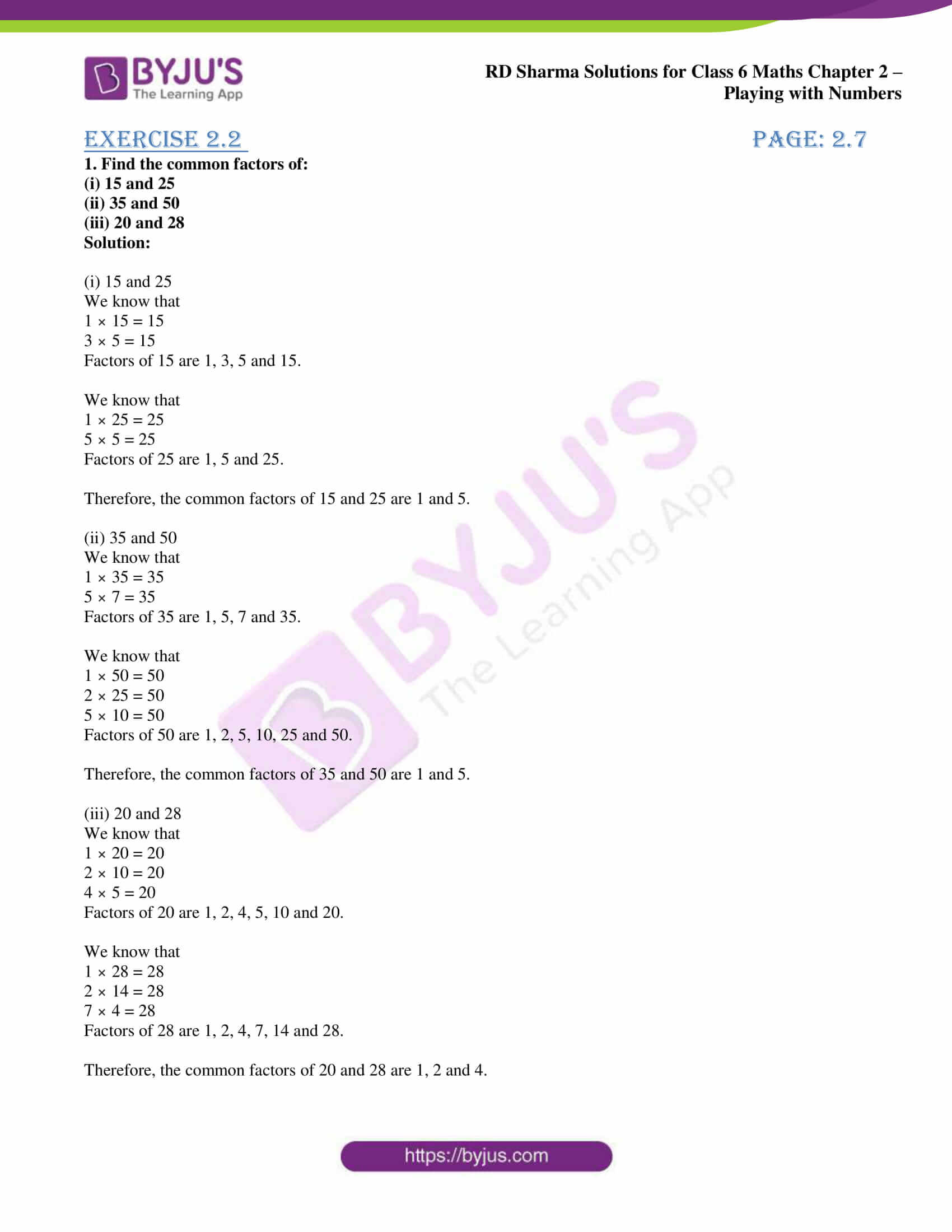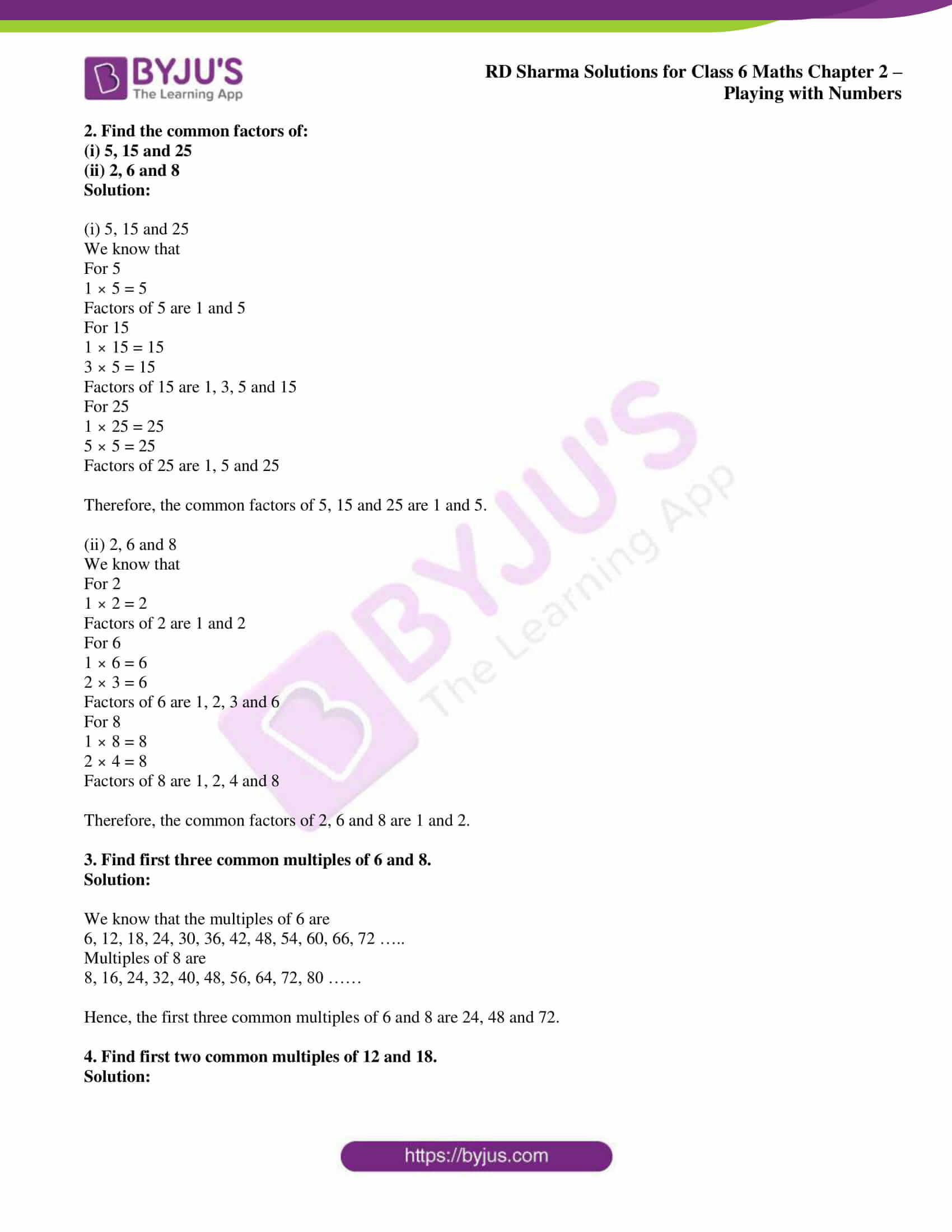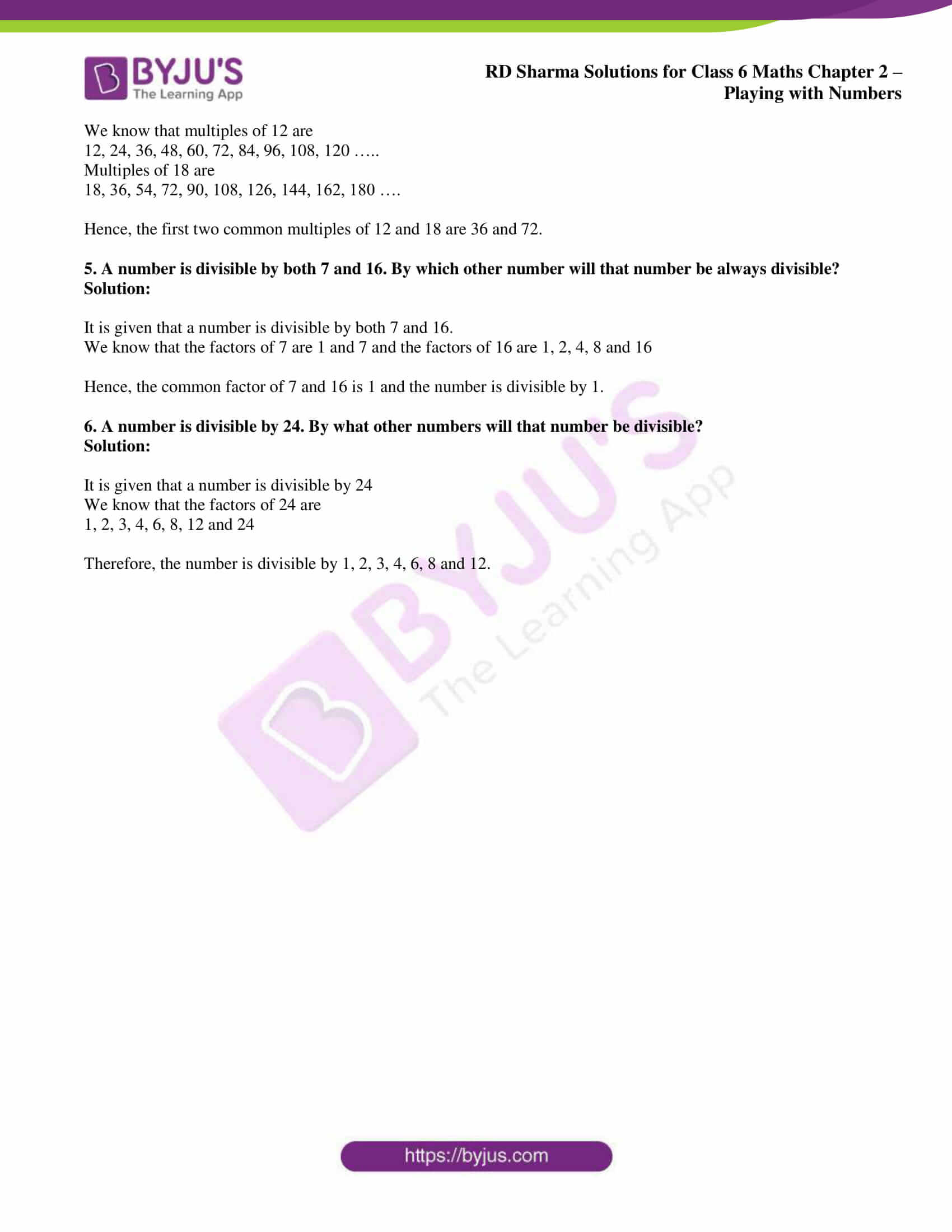# RD Sharma Solutions for Class 6 Maths Chapter 2: Playing with Numbers Exercise 2.2

## RD Sharma Solutions for Class 6 Maths Exercise 2.2 PDF

The subject faculty at BYJU’S creates chapter wise solutions with explanations in a simple language which matches the understanding capacity of students. RD Sharma Solutions PDF can be used by the students as a reference guide which helps them understand the various methods of solving problems. The important formulas and shortcuts are highlighted in order to help students grasp them faster. The stepwise and detailed solutions provided for each exercise help students to cover the entire syllabus accordingly. RD Sharma Solutions for Class 6 Maths Chapter 2 Playing with Numbers Exercise 2.2 are provided here.

## RD Sharma Solutions for Class 6 Maths Chapter 2: Playing with Numbers Exercise 2.2 Download PDF## Access answers to Maths RD Sharma Solutions for Class 6 Chapter 2: Playing with Numbers Exercise 2.2

1. Find the common factors of:

(i) 15 and 25

(ii) 35 and 50

(iii) 20 and 28

Solution:

(i) 15 and 25

We know that

1 × 15 = 15

3 × 5 = 15

Factors of 15 are 1, 3, 5 and 15.

We know that

1 × 25 = 25

5 × 5 = 25

Factors of 25 are 1, 5 and 25.

Therefore, the common factors of 15 and 25 are 1 and 5.

(ii) 35 and 50

We know that

1 × 35 = 35

5 × 7 = 35

Factors of 35 are 1, 5, 7 and 35.

We know that

1 × 50 = 50

2 × 25 = 50

5 × 10 = 50

Factors of 50 are 1, 2, 5, 10, 25 and 50.

Therefore, the common factors of 35 and 50 are 1 and 5.

(iii) 20 and 28

We know that

1 × 20 = 20

2 × 10 = 20

4 × 5 = 20

Factors of 20 are 1, 2, 4, 5, 10 and 20.

We know that

1 × 28 = 28

2 × 14 = 28

7 × 4 = 28

Factors of 28 are 1, 2, 4, 7, 14 and 28.

Therefore, the common factors of 20 and 28 are 1, 2 and 4.

2. Find the common factors of:

(i) 5, 15 and 25

(ii) 2, 6 and 8

Solution:

(i) 5, 15 and 25

We know that

For 5

1 × 5 = 5

Factors of 5 are 1 and 5

For 15

1 × 15 = 15

3 × 5 = 15

Factors of 15 are 1, 3, 5 and 15

For 25

1 × 25 = 25

5 × 5 = 25

Factors of 25 are 1, 5 and 25

Therefore, the common factors of 5, 15 and 25 are 1 and 5.

(ii) 2, 6 and 8

We know that

For 2

1 × 2 = 2

Factors of 2 are 1 and 2

For 6

1 × 6 = 6

2 × 3 = 6

Factors of 6 are 1, 2, 3 and 6

For 8

1 × 8 = 8

2 × 4 = 8

Factors of 8 are 1, 2, 4 and 8

Therefore, the common factors of 2, 6 and 8 are 1 and 2.

3. Find first three common multiples of 6 and 8.

Solution:

We know that the multiples of 6 are

6, 12, 18, 24, 30, 36, 42, 48, 54, 60, 66, 72 …..

Multiples of 8 are

8, 16, 24, 32, 40, 48, 56, 64, 72, 80 ……

Hence, the first three common multiples of 6 and 8 are 24, 48 and 72.

4. Find first two common multiples of 12 and 18.

Solution:

We know that multiples of 12 are

12, 24, 36, 48, 60, 72, 84, 96, 108, 120 …..

Multiples of 18 are

18, 36, 54, 72, 90, 108, 126, 144, 162, 180 ….

Hence, the first two common multiples of 12 and 18 are 36 and 72.

5. A number is divisible by both 7 and 16. By which other number will that number be always divisible?

Solution:

It is given that a number is divisible by both 7 and 16.

We know that the factors of 7 are 1 and 7 and the factors of 16 are 1, 2, 4, 8 and 16

Hence, the common factor of 7 and 16 is 1 and the number is divisible by 1.

6. A number is divisible by 24. By what other numbers will that number be divisible?

Solution:

It is given that a number is divisible by 24

We know that the factors of 24 are

1, 2, 3, 4, 6, 8, 12 and 24

Therefore, the number is divisible by 1, 2, 3, 4, 6, 8 and 12.

### RD Sharma Solutions for Class 6 Maths Chapter 2 – Playing with Numbers Exercise 2.2

RD Sharma Solutions Class 6 Maths Chapter 2 Playing with Numbers Exercise 2.2 explains the method of finding common factors and multiples for the given set of questions.

### Key features of RD Sharma Solutions for Class 6 Maths Chapter 2: Playing with Numbers Exercise 2.2

• Students can improve their problem solving abilities with the help of RD Sharma Solutions.
• The solutions are available both exercise wise and chapter wise based on the requirement of students.
• Time taken in solving complex problems is reduced as the students have better conceptual knowledge.
• The objective of creating exercise wise solutions is to help students perform well in the exam.